Processing ......FreeComputerBooks.com Links to Free Computer, Mathematics, Technical Books all over the World

Algebra: A Classical Approach
Top Free Machine Learning Books 🌠 - 100% Free or Open Source!
• Title Algebra: A Classical Approach
• Author(s) Jon Blakely
• Publisher: JonBlakely.com (2021 - Date)
• Hardcover/Paperback N/A
• eBook PDF Files
• Language: English
• ISBN-10: N/A
• ISBN-13: N/A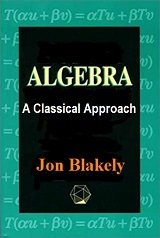Book Description

This book provides a complete and contemporary perspective on classical polynomial algebra through the exploration of how it was developed and how it exists today. It facilitates a thorough understanding of algebra and illustrates how the concepts of modern algebra originally developed from classical algebraic precursors.

It will facilitate skills in algebra. Inside are numerous lessons to assist you better understand the topic. These lessons are among many exercises to practice what you’ve learned, together with a whole answer key to test your work

It also provides content focused on key topics only, with discrete explanations of critical concepts taught in a typical Algebra course, from functions and FOILs to quadratic and linear equations.

Throughout this book, you'll learn the terms to assist you understand algebra, and you'll expand your knowledge of the topic through dozens of sample problems and their solutions. With the teachings during this book, you'll find it easier than ever to understand concepts in algebra.

• Jon Blakely is a Full-Time Tenured Instructor at Department of Mathematics at College of the Sequoias.
Reviews, Ratings, and Recommendations: Related Book Categories: Read and Download Links:Similar Books:
•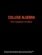College Algebra (Jay Abramson, et al.)

This is a book on linear algebra and matrix theory. It gives a self- contained treatment of linear algebra with many of its most important applications which does not neglect arbitrary fields of scalars and the proofs of the theorems.

•Linear Algebra (Jim Hefferon)

This textbook covers linear systems and Gauss' method, vector spaces, linear maps and matrices, determinants, and eigenvectors and eigenvalues. Each chapter has three or four discussions of additional topics and applications.

•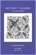Abstract Algebra: Theory and Applications (Thomas W Judson)

This book is designed to teach the principles and theory of abstract algebra to college juniors and seniors in a rigorous manner. Its strengths include a wide range of exercises, both computational and theoretical, plus many nontrivial applications.

•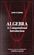Algebra: A Computational Introduction (John Scherk)

This book is a unique approach and presentation, the author demonstrates how software can be used as a problem-solving tool for algebra. It includes many computations, both as examples and as exercises.

•A Computational Introduction to Number Theory and Algebra

This introductory book emphasises algorithms and applications, such as cryptography and error correcting codes, and is accessible to a broad audience. It covers the basics of number theory, abstract algebra and discrete probability theory.

•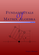Fundamentals of Matrix Algebra (Gregory Hartman)

A college (or advanced high school) level text dealing with the basic principles of matrix and linear algebra. It covers solving systems of linear equations, matrix arithmetic, the determinant, eigenvalues, and linear transformations.

•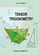Tensor Trigonometry (A.S. Ninul)

The tensor trigonometry is development of the flat scalar trigonometry from Leonard Euler classic forms into general multi-dimensional tensor forms with vector and scalar orthoprojections and with step by step increasing complexity and opportunities.

•Computational Linear Algebra and N-dimensional Geometry

This undergraduate textbook on Linear Algebra and n-Dimensional Geometry, in a self-teaching style, is invaluable for sophomore level undergraduates in mathematics, engineering, business, and the sciences.

•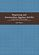Beginning and Intermediate Algebra (Tyler Wallace)

These books use a teacherly writing style and a careful blend of skills development and conceptual questions to meet the unique needs of the developmental math student. It takes advantage of experiences in the classroom and an editing eye to offer one of the most well-rounded series available, written with the developmental learner in mind.

•Modeling, Functions, and Graphs: Algebra for College Students

This book includes content found in a typical algebra course, along with introductions to curve-fitting and display of data. The authors focus on three core themes throughout their textbook: Modeling, Functions, and Graphs.

•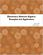Elementary Abstract Algebra: Examples and Applications

It introduces the basic notions of group theory by a thorough treatment of important examples, including complex numbers, modular arithmetic, symmetries, and permutations, also applications to communications, cryptography, and coding theory.

•Advanced Algebra (Anthony W. Knapp)

This book is on modern algebra which treat various topics in commutative and noncommutative algebra and provide introductions to the theory of associative algebras, homological algebras, algebraic number theory, and algebraic geometry.

•Basic Algebra (Anthony W. Knapp)

This book is on Preliminaries about the Integers, Polynomials, and Matrices; Vector Spaces over Q, R, and C; Inner-Product Spaces; Groups and Group Actions; Theory of a Single Linear Transformation; Multilinear Algebra; Advanced Group Theory; etc.

Book Categories
 :All CategoriesTop Free BooksRecent BooksMiscellaneous BooksComputer EngineeringComputer LanguagesComputer ScienceData Science/DatabasesJava and Java EE (J2EE)Linux and UnixMathematicsMicrosoft and .NETMobile ComputingNetworking and CommunicationsSoftware EngineeringSpecial TopicsWeb Programming
Other Categories